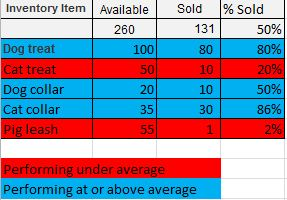# QlikView App Dev

Discussion Board for collaboration related to QlikView App Development.

Announcements
See why Qlik was recognized for the seventh year in a row – and discover how we can help you tackle your data integration challenges. Get the report
cancel
Showing results for
Did you mean:Not applicable

## Color background color based on column partial sum

Using pivot tables, how do I do the following.So, I want to color code the row depending on how it's % Sold is doing compared to the total average (partial sum)

So, for example, Cat Treat and Pig Leash have only sold 20% and 2% respectively while the overall average is 50% for all products, so it gets red.

So, I know I need something like this for my background colors........

if(total for column 3 >= column 3 for item, RED, BLUE)

Thank you so much.

1 Solution

Accepted SolutionsMVP

I think something like sum(total column(2) ) will not work.

Try

=if( sum(sold_count) / sum(available_count) >= sum(total sold_count)/sum(total available_count), red(), blue())

7 RepliesMVP

if % Sold is maybe calculated like

=sum(Sold) / sum(Available)

then your background color expression may look like

=if( sum(Sold) / sum(Available) >= sum(total Sold) / sum(total Available), red(), blue() )

Hope this helps,

StefanNot applicable
Author

Kind of.

Here are the defs:

column(1) = Available = sum(available_count)

column(2) = Sold = sum(sold_count)

column(3) = % Sold = column(2) / column(1)

So, I went into the background color and put this in but it's not working as expected.  It's all blue.

=if(column(2)/column(1) >= sum(total column(2)) / sum(total column(1)), red(), blue())MVP

I think something like sum(total column(2) ) will not work.

Try

=if( sum(sold_count) / sum(available_count) >= sum(total sold_count)/sum(total available_count), red(), blue())Not applicable
Author

Thank you.  That works!

I can start another post but I just tried making the pivot table a little more multi-dimensional by adding Sale Year to it, so now it looks like this:Using the formula above worked when it wasn't by year but now it appear the TOTAL portion of that expression totals all columns up?  So, since the overall percentage is 53% (131+61/260+100), Dog collar in 2011 is showing up RED since it's below 53% but really it should be blue because it's above 50% for that specific year.  Does that make sense?

Is there a way to get the partial sum for the column instead of the total sum for all the columns?Not applicable
Author

I think I figured it out.  Need to add the column you want to group by after the total, so this:

=if( sum(sold_count) / sum(available_count) >= sum(total <TheYear> sold_count)/sum(total <TheYear> available_count), red(), blue())

Right?MVP

Yes, should be correct. You can list the fields to ignore in the total, so you can still group by, using the <> syntax.Creator II